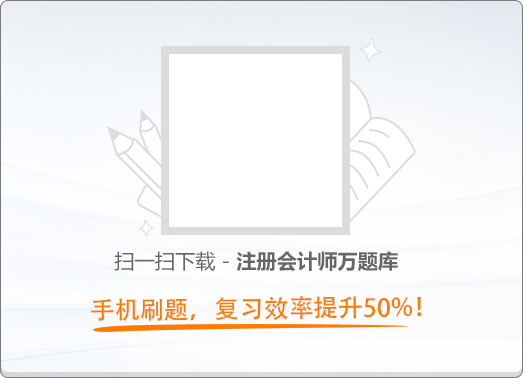150:002018年注册会计师《公司战略与风险管理》真题

1
(单项选择题)

• A.

导入期

• B.

成长期

• C.

成熟期

• D.

衰退期

• A
• B
• C
• D

2
(单项选择题)

• A.

N国电动汽车市场刚刚兴起，市场需求增长较快

• B.

N国电动汽车零部件市场比较落后，供应商管理水平较低

• C.

N国劳动力价格相对C国较低，工人技术水平和文化素质不高

• D.

N国政府为了保护本国汽车产业，对甲公司的进入设定了限制条件

• A
• B
• C
• D

3
(单项选择题)

• A.

物理上独特的资源

• B.

具有经济制约性的资源

• C.

具有路径依赖性的资源

• D.

具有因果含糊性的资源

• A
• B
• C
• D

4
(单项选择题)

• A.

以企业使命为基础

• B.

加强协调作用

• C.

根据文化的要求进行管理

• D.

重新制定战略

• A
• B
• C
• D

5
(单项选择题)

• A.

权力导向型

• B.

人员导向型

• C.

角色导向型

• D.

任务导向型

• A
• B
• C
• D

6
(单项选择题)

• A.

增长型战略

• B.

扭转型战略

• C.

多种经营战略

• D.

防御型战略

• A
• B
• C
• D

7
(单项选择题)

• A.

技术设计风险

• B.

技术选择风险

• C.

技术研发风险

• D.

技术应用风险

• A
• B
• C
• D

8
(单项选择题)

M国某地区位于地震频发地带，那里的居民具有较强的防震意识，住房普遍采用木制结构，抗震性能优越。不少家庭加装了地震时会自动关闭煤气的仪器，以防范地震带来的相关灾害。根据上述信息，该地区居民采取的风险管理策略工具是（　）。

• A.

风险转移

• B.

风险控制

• C.

风险转换

• D.

风险规避

• A
• B
• C
• D

9
(单项选择题)

• A.

造船业务

• B.

港口建设业务

• C.

海运业务

• D.

相关智能设备制造业务

• A
• B
• C
• D

10
(单项选择题)

• A.

国际战略

• B.

多国本土化战略

• C.

全球化战略

• D.

跨国战略

• A
• B
• C
• D

11
(单项选择题)

• A.

市场开发战略

• B.

差异化战略

• C.

集中化战略

• D.

多元化战略

• A
• B
• C
• D

12
(单项选择题)

• A.

战略性

• B.

专业性

• C.

二重性

• D.

系统性

• A
• B
• C
• D

13
(单项选择题)

• A.

生产管理

• B.

营销管理

• C.

成本管理

• D.

市场调研与预测

• A
• B
• C
• D

14
(单项选择题)

• A.

运营风险

• B.

市场风险

• C.

产业风险

• D.

财务风险

• A
• B
• C
• D

15
(单项选择题)

• A.

寻求支配地位

• B.

不能保持严格的战略约束力

• C.

过分集权化

• D.

不了解竞争者的战略目标和管理费用

• A
• B
• C
• D

16
(单项选择题)

• A.

个人的素质和影响

• B.

在管理层次中的地位

• C.

利益相关者集中或联合的程度

• D.

参与或影响企业战略决策与实施过程

• A
• B
• C
• D

17
(单项选择题)

• A.

连续阶段

• B.

渐进阶段

• C.

不断改变阶段

• D.

全面阶段

• A
• B
• C
• D

18
(单项选择题)

• A.

事业部制组织结构

• B.

M型组织结构

• C.

职能制组织结构

• D.

创业型组织结构

• A
• B
• C
• D

19
(单项选择题)

• A.

该方法的优点是简单明了易于操作

• B.

该方法的使用效果依赖于专业人员的水平

• C.

该方法适用于组织规模较小、流程较简单的业务风险分析

• D.

该方法可以对企业生产或经营中的风险及其成因进行定性分析

• A
• B
• C
• D

20
(单项选择题)

• A.

技术风险

• B.

财务风险

• C.

战略风险

• D.

产业风险

• A
• B
• C
• D

21
(单项选择题)

2016年以来，生产安保设施的天盾公司先后收购了两家同类企业，在扩大生产经营规模、降低成本的同时，开发出功能优于其他同类产品的新产品。天盾公司的上述收购行为属于该公司的（　）。

• A.

总体战略

• B.

业务单位战略

• C.

职能战略

• D.

混合战略

• A
• B
• C
• D

22
(单项选择题)

• A.

防御型战略组织

• B.

开拓型战略组织

• C.

分析型战略组织

• D.

反应型战略组织

• A
• B
• C
• D

23
(单项选择题)

• A.

“防御者”战略

• B.

“扩张者”战略

• C.

“躲闪者”战略

• D.

“抗衡者”战略

• A
• B
• C
• D

24
(单项选择题)

• A.

对抗

• B.

和解

• C.

折中

• D.

协作

• A
• B
• C
• D